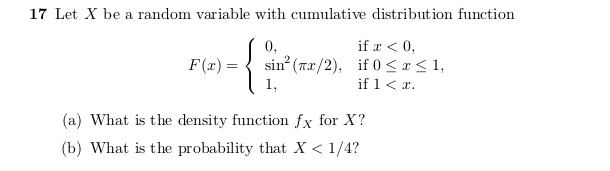1. The density function $$f_X$$ for X can be computed by taking the derivative of the

cumulative distribution function $$F(X)$$

The derivative of a constant is 0 so we will only differentiate the function

$$d/dx(F(X)) = d/dx(sin^2(\pi*x/2))$$ for $$0 \le x \le 1$$

We could use the chain rule to differentiate the function or use a trignometric identity:

• $$sin^2(\theta) = (1-cos(2\theta)) / 2$$

to make it easier.

so $$d/dx(1/2 - cos(2(\pi x/2)) = d/dx\big(1/2 - cos(\pi x) / 2\big) =$$

$$\pi sin(\pi x)/2 = f_X$$

1. Finding the probably we have to use the cumulative distribution function F(X)

and compute integrals.

For the probability that X < 1/4, we compute

$$\int_{0}^{1/4} sin^2(\pi x/2) dx$$

we use the trig identity again to make integrating the function easier

$$1/2\int_{0}^{1/4} (1 - cos(\pi x)) dx = 1/2 \bigg[x - sin(\pi x)/ \pi \bigg]_{0}^{1/4} =$$

$$1/2\bigg(1/4 - sin(\pi/4)/ \pi\bigg) = 1/8 - \sqrt{2}/4 \pi$$